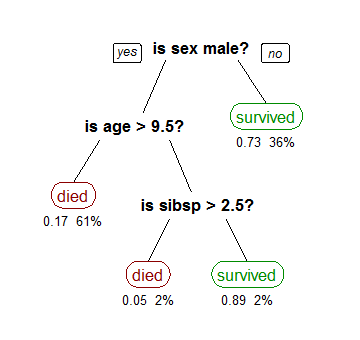# Using Decision Trees to predict infant birth weightsIn this article, I will show you how to use decision trees to predict whether the birth weights of infants will be low or not. We will use the birthwt data from the MASS library.

What is a decision tree?

A decision tree is an algorithm that builds a flowchart like graph to illustrate the possible outcomes of a decision. To build the tree, the algorithm first finds the variable that does the best job of separating the data into two groups. Then, it repeats the above step with the other variables.

This results in a tree graph, where each split represents a decision. The algorithm chooses the splits such that the maximum number of observations are classified correctly. The biggest advantage of a decision tree is that it is really intuitive and can be understood even by people with no experience in the field.

For example, a classification tree showing the survival of passengers of the Titanic is as follows (source: Wikipedia):## Share:

Subscribe
Notify ofInline Feedbacks

On social media